# CBSE Worksheet for chapter-7 Lines and Angles class 9

## Find CBSE Worksheet for chapter-7 Lines and Angles class 9

CLASS-9

BOARD: CBSE

Mathematic Worksheet - 7

TOPIC: Lines and Angles

This page is prepared by the Academic team of Physics Wallah which consists of CBSE Board Worksheet for Class 9 Maths. Students of Class 9 Maths can get a free Worksheet for Class 9 Maths in PDF format prepared as per the newest syllabus and examination pattern in your schools.

Before solving the Worksheet for Class 9 one must read the textbook of your school board exam and try to read the notes given in Physics Wallah Class 9 notes sections. Students can also download free pdf of Class 9 Maths Notes prepared by teachers and solve important problems provided here with solutions on daily basis to get more scores in school exams and tests. Physics Wallah worksheets for Class 9 CBSE can help students practice with tests and quickly revise lessons learned in the classroom with essential resources such as video lessons, short notes, and sample papers.

Class 9 Board is one of the most important year for every student and need a good planning and right study material to score good marks in your final exam. For other CBSE Worksheets for class 9 Mathematics check out the main page of Physics Wallah.

## Summary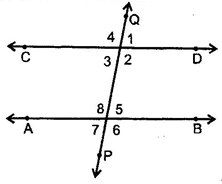1. Transversal: A line which intersects two or more given parallel lines at distinct points is called a transversal of the given lines.
2. Corresponding angles: 1 & 5, 4 & 8, 2 & 6, 3 & 7 are pairs of corresponding angles.
3. Alternate interior angles: 3 & 5, 2 & 8 are the pairs of alternate interior angles.
4. Consecutive interior angles: 2 & 5, 3 & 8, are pairs of consecutive interior angles.
5. Vertically opposite angles: in figure, 1 = 3, 2 = 4, 5 = 7 & 6 = 8.

### Objective- 1

Q1. Two parallel lines have

1. a common point
2. two common points
3. no common point
4. infinite common points

Q2. An angle is 14o more than its complementary angle then angle is

1. 38o
2. 52o
3. 50o
4. None of these

Q3. X lies in the interior of BAC. If ∠BAC=70oand ∠BAX=42o , ∠CAX=

1. 280
2. 290
3. 270
4. 300

Q4. Two angles whose measure are a and b are such that 2a-3b=600 then =?, if they form a linear pair

1. 0
2. 8/5
3. 1/2
4. 2/3

Q5. Which one of the following is correct?

1. If two parallel lines are intersected by a transversal, then alternate angles are equal.
2. If two parallel lines are intersected by a transversal, then sum of the interior angles on the same side of transversal is 180o
3. If two parallel lines are intersected by a transversal, then corresponding angles are equal
4. All of these.

### Objective- 2

Q1. Two supplementary angles are in ratio 4 : 5, then the angles are:

1. 80°, 100°
2. 70°, 110°
3. 80°, 120°
4. None

Q2. If an angle differs from its complement by 10°, find the larger angle.

1. 45°
2. 50°
3. 95°
4. 60°

Q3. In the given figure, OP and OQ bisect BOC and AOC respectively, then POQ is.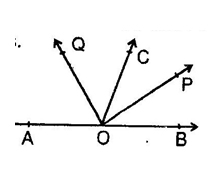1. 90°
2. 45°
3. 135°
4. 180°

Q4. In the given fig if l II m, n II p and 1 = 85° then 2 is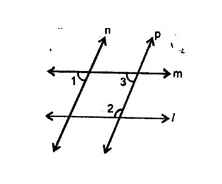1. 95°
2. 110°
3. 135°
4. 85°

Q5. An larger angle is 14° more than its complementary angle then angle is:

1. 38°
2. 52°
3. 50°
4. none of these

Q6. X lies in interior of ∠BAC. If ∠BAC = 70° and ∠BAX = 42° then ∠XAC = ?

1. 28°
2. 29°
3. 27°
4. 30°

Q7. If the supplement of an angle is three times its complement, then angle is:

1. 40°
2. 35°
3. 50°
4. 45°

Q8. Two angles whose measures are a & b are such that 2a – 3b = 60°, if they form a linear pair then find .

1. 0
2. 8/5
3. 1/2
4. 2/3

Q9. The supplement of an angle is one third of itself. Then the angle is:

1. 135°
2. 45°
3. 140°
4. None

Q10. In given figure, if ∠BOC = 7x + 20° and ∠COA = 3x, then the value of x for which AOB becomes a straight line.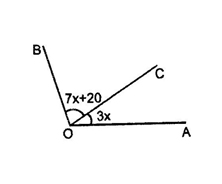1. 15°
2. 12°
3. 16°
4. None

Q11. In given figure if AB II DF, AD II FG ∠BAC = 65°, ∠ACB=55o ∠FGH is: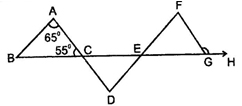1. 120°
2. 125°
3. 115°
4. 140°

Q12. In given figure, AB II ED and ∠ABC = 30°, ∠EDC = 70° then x is: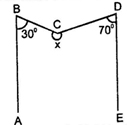1. 240°
2. 125°
3. 260°
4. None

Q13. In the given figure, if EC II AB, ∠ECD = 70° and ∠BDO = 20°, then ∠OBD is-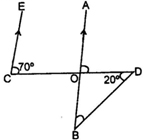1. 20°
2. 50°
3. 60°
4. 70°

Q14. Given AB II CD, x° =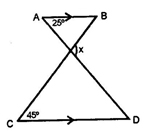1. 20°
2. 70°
3. 110°
4. 35°

Q15. As shown in the figure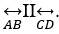. If point P is between these two lines such that m∠ABP = 50° and m∠CDP = 70° then find ∠BPD.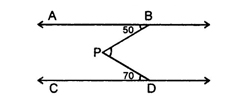1. 120°
2. 70°
3. 110°
4. 50°

Q16. The measure of that angle which is four times its supplement is:

1. 36
2. 144°
3. 155°
4. 180°

1. 3
2. 2
3. 1
4. 2
5. 4

1. 1
2. 2
3. 1
4. 1
5. 2
6. 1
7. 4
8. 2
9. 1
10. 3
11. 2
12. 3
13. 2
14. 2
15. 1
16. 2